# RL — LQR & iLQR Linear Quadratic Regulator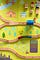Photo by Jason Leung

Reinforcement learning can be divided into Model-free and Model-based learning. Model-free learning emphasizes heavily on sampling. It collects a large number of training samples to fit the policy or the value function without knowing how things work. Model-based learning, on the contrary, develops a model (the system dynamics) to optimize controls. Mathematically, the model f predicts the next state when taken an action u from a state x.

In this article, we will look into two commonly used trajectory optimization methods using a known or a learned system dynamics. Linear Quadratic Regulator LQR and iLQR calculate an optimal trajectory from the initial to the target state by optimizing a cost function.

LQR assumes the model is locally linear. iLQR uses an iterative version of LQR to find the optimal trajectory for non-linear systems. These methods are frequently used in many model-based methods. So it worths some time to study it. Be warned that the equations are tedious. But once past the fear and with some patience, the principle is really not hard.

LQR

LQR assumes the model is locally linear and time-varied, and we represent it in a matrix form as:

We also define a time-varied cost function to find the optimal trajectory that obeys the model. Mathematically, our objective is:

Therefore, if the system dynamics f (model) and the cost function c is known, we can plan our optimal controls (actions) using a trajectory optimization method.

Example

Let’s demonstrate the states, actions, and models with some simple examples. Our state may compose of the location and the velocity in the x and y-direction of an object.

Our actions are the forces applied.

The objective below minimizes the amount of force applied.

But this is not very interesting. We can add another term to penalize the system if it deviates from a planned trajectory.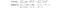Source

The following is an example of the possible model.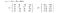Source

# Recap

The following are two different paths with different actions.

Our problem statement is:

# Linear Quadratic Regulator LQR Overview

Let’s have an overview of the algorithm first. Otherwise, we may get lost easily.

In LQR,

• We take a backward pass from the target state to the initial state. We optimize the cost at each time step, so we can express the optimal action in terms of the state.
• Once the backward pass reaches the initial state, we know x1 and therefore, we can find the first action u1 to be taken.
• Last we perform a forward pass which we use the model to calculate the next state and use the equation in the backward pass to find the next optimal action.

Backward and forward path

Let’s run through the backward pass and the forward pass quickly again. In the backward path, we calculate the Q-value function using

We optimize Q, so we can express the optimal action in terms of the state and compute the values for K and k.

As we move backward, we reach the initial state x1 which is known and therefore, we can finally find u1.

The forward path is very simple. We use the model f to compute the next state. And use the optimized equation to find the action.

Here is the big picture. Once we get it, the detail will look much simpler.

# Backward pass

The backward pass is the most complicated and can get lost easily. It involves equations not targeted for the human consumption. But we will group that properly to make the points clear and manageable.

Time step T

Let’s start with the last time step in the backward pass.

Our prime objective here is to express the optimal action in terms of the state and compute the value for K and k.

The cost function at time step t for the current actions is defined as:

We are going to break C into four sub-matrices and c into two so the equations will look more manageable later.

Cxx composes of the coefficient in C that will multiply x, Cxx, and x together.

Cux composes of the coefficient in C that will multiply u, Cux, and x together etc

We generalize the cost function a little bit more by adding a constant and name it Q (a.k.a. Q-value function). We take the derivative to optimize the actions. As shown, we can express the optimal action in terms of the current state. And K and k can be computed using the sub-matrices of C.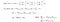Source

Here, we reach the first milestone:

Compute V

Now we can express u in terms of x.

We can plug u it into Q.

Q is now no longer depend on u: the L.H.S. Q(x, x) above actually becomes the value function V(x). Now with some heavy regrouping below, we can express V in terms of x, C, K, and k. This will be needed in the next step.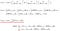Source

Time step T-1

Now, we move one more step backward.

We will compute Q again. But there is a major difference here. T-1 is not a terminal state and therefore we need to add the value function for the next state back.

i.e.

And we just compute V from our last section. Apply the previous result,

and the model

We get,

Grouping terms again, we can simplify the equation to: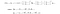Source

Optimize Q again: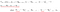Source

Now, this is the major milestone of expressing the action in terms of the current state by minimizing the cost.

As we continue moving backward, we reach the initial state x1 which is known. And therefore, we can compute the first action u1.

# Forward pass

The forward pass is very simple and just repeat the step below until reaching the target state.

Time step 1

In the backward pass, we compute the K and k when optimizing Q.

Now, using the current state, we can compute the action from

With both current action and state, we can find the next state with the model.

LQR algorithm

Congratulations and thanks for the patience! Here is the final algorithm.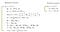Source

# Stochastic dynamics

Can we use LQR if the model is stochastic? Actually, we can use LQR as if the model is deterministic.

A deterministic dynamics is defined as:

We can model the stochastic dynamics with a Gaussian distribution:

The good news is that we can still apply LQR with action taken as:

We can ignore Σ as it will cancel others out.

# Iterative Linear Quadratic Regulator iLQR for Non-linear models

In LQR, we assume the model is locally linear. iLQR expands LQR to non-linear models. iLQR actually reuses LQR iterative to refine the trajectory. In iLQR, we start with a guessing trajectory and run LQR to refine the guess. We continue refining the guess iteratively with LQR and eventually, it will converge to the optimal solution.

Newton’s method for optimization

This process is very similar to Newton’s method for optimization. Gradient Descent uses the first-order derivative f’ to approximate a function and use it to locate the next guess for the optimal point. Newton’s method is more accurate and converges faster by using both the first-order and second-order derivative to create a quadratic approximation of the original function f.

Starting with an initial guess, we construct a quadratic approximation to f. We optimize the quadratic function and use its optimal point as the next guess which is computed as:

Eventually, the guess will converge to the optimal point of f if it exists.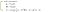Source

In LQR, the dynamic model is linear. iLQR expands the concept to non-linear models. We approximate the dynamic model to first order and the cost function to the second order:

Rearrange the terms a little bit,

We can reformulate the equations as: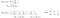Modified from source

These equations look almost the same as the equations used in LQR:

Instead of taking x and u as the input parameters, the new functions take δx and δu.

Here, we want to find the δx and δu which locates the optimal point of the approximated function. To do that, we use LQR as the inner loop optimization to find the new guess.

iLQR algorithm

Here is the algorithm: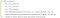Source

In short, iLQR is an approximation of Newton’s method for solving:

Differential dynamic programming DDP

The iLQR method does not expand the dynamics to the second order so it is not exactly like Newton’s method. If we actually include the second term, this becomes Newton’s optimization and it is called DDP.

DDP is for the completion of our discussion. For the rest of our model-based RL discussion, we will mainly focus on iLQR.

Iterative LQR with Line Search

However, iLQR may have an overshoot problem just like the Newton’s method. If the original function is highly curved, a second order approximation may not be accurate enough. The optimal point of the approximation may overshoot the real optimal point.

To correct that, we can apply a line search (for example, a binary search) between the original guess and the new guess to locate the optimal point within this line search. i.e. we can apply α below with values ranging from 0 to 1 to search for the lowest point in the line search to avoid overshoot.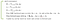Source

# Thoughts

iLQR and LQR form the fundamental tools for model-based reinforcement learning. Combining the knowledge of the model and the cost function, we can plan the optimal actions accordingly. The equations may be tedious but we hope the explanations here will be it easier.

# Credits & references

The proof in this article is based on UC Berkely Reinforcement Learning course in the optimal control and planning.

Written by

## Jonathan Hui

#### Deep Learning

Welcome to a place where words matter. On Medium, smart voices and original ideas take center stage - with no ads in sight. Watch
Follow all the topics you care about, and we’ll deliver the best stories for you to your homepage and inbox. Explore
Get unlimited access to the best stories on Medium — and support writers while you’re at it. Just \$5/month. Upgrade